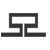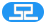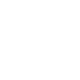# wordpress的发布时间显示为发布于xx之前

``````while (have_posts()) : the_post();
echo '发布于' . human_time_diff( get_the_time( 'U' ), current_time( 'timestamp' ) ) . ' 前';
endwhile;``````

``````function human_time_diff( \$from, \$to = 0 ) {
if ( empty( \$to ) ) {
\$to = time();
}

\$diff = (int) abs( \$to - \$from );

if ( \$diff < MINUTE_IN_SECONDS ) {
\$secs = \$diff;
if ( \$secs <= 1 ) {
\$secs = 1;
}
/* translators: Time difference between two dates, in seconds. %s: Number of seconds. */
\$since = sprintf( _n( '%s second', '%s seconds', \$secs ), \$secs );
} elseif ( \$diff < HOUR_IN_SECONDS && \$diff >= MINUTE_IN_SECONDS ) {
\$mins = round( \$diff / MINUTE_IN_SECONDS );
if ( \$mins <= 1 ) {
\$mins = 1;
}
/* translators: Time difference between two dates, in minutes (min=minute). %s: Number of minutes. */
\$since = sprintf( _n( '%s min', '%s mins', \$mins ), \$mins );
} elseif ( \$diff < DAY_IN_SECONDS && \$diff >= HOUR_IN_SECONDS ) {
\$hours = round( \$diff / HOUR_IN_SECONDS );
if ( \$hours <= 1 ) {
\$hours = 1;
}
/* translators: Time difference between two dates, in hours. %s: Number of hours. */
\$since = sprintf( _n( '%s hour', '%s hours', \$hours ), \$hours );
} elseif ( \$diff < WEEK_IN_SECONDS && \$diff >= DAY_IN_SECONDS ) {
\$days = round( \$diff / DAY_IN_SECONDS );
if ( \$days <= 1 ) {
\$days = 1;
}
/* translators: Time difference between two dates, in days. %s: Number of days. */
\$since = sprintf( _n( '%s day', '%s days', \$days ), \$days );
} elseif ( \$diff < MONTH_IN_SECONDS && \$diff >= WEEK_IN_SECONDS ) {
\$weeks = round( \$diff / WEEK_IN_SECONDS );
if ( \$weeks <= 1 ) {
\$weeks = 1;
}
/* translators: Time difference between two dates, in weeks. %s: Number of weeks. */
\$since = sprintf( _n( '%s week', '%s weeks', \$weeks ), \$weeks );
} elseif ( \$diff < YEAR_IN_SECONDS && \$diff >= MONTH_IN_SECONDS ) {
\$months = round( \$diff / MONTH_IN_SECONDS );
if ( \$months <= 1 ) {
\$months = 1;
}
/* translators: Time difference between two dates, in months. %s: Number of months. */
\$since = sprintf( _n( '%s month', '%s months', \$months ), \$months );
} elseif ( \$diff >= YEAR_IN_SECONDS ) {
\$years = round( \$diff / YEAR_IN_SECONDS );
if ( \$years <= 1 ) {
\$years = 1;
}
/* translators: Time difference between two dates, in years. %s: Number of years. */
\$since = sprintf( _n( '%s year', '%s years', \$years ), \$years );
}

/**
* Filters the human readable difference between two timestamps.
*
* @since 4.0.0
*
* @param string \$since The difference in human readable text.
* @param int    \$diff  The difference in seconds.
* @param int    \$from  Unix timestamp from which the difference begins.
* @param int    \$to    Unix timestamp to end the time difference.
*/
return apply_filters( 'human_time_diff', \$since, \$diff, \$from, \$to );
}``````

## 相关文章:兼容浏览器

## 热销主题### 旅游、景点主题wordpress主题7折

¥799### 通用设备主题7折

¥799### 家具公司wordpress主题7折

¥799### 盆栽花店wordpress主题7折

¥799#### 7*12服务咨询电话：

133-7205-6573